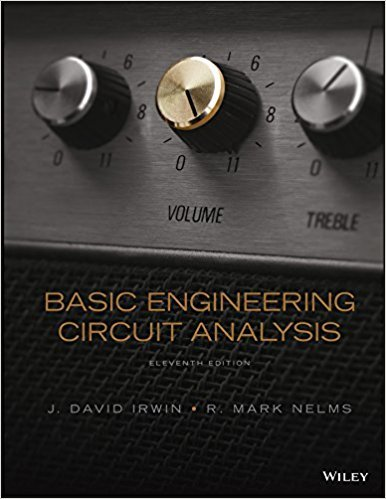×
Get Full Access to Basic Engineering Circuit Analysis - 11 Edition - Chapter 6 - Problem 6.14
Get Full Access to Basic Engineering Circuit Analysis - 11 Edition - Chapter 6 - Problem 6.14

×

# The waveform for the current flowing through the 10-FISBN: 9781118539293 159

## Solution for problem 6.14 Chapter 6

Basic Engineering Circuit Analysis | 11th Edition

• Textbook Solutions
• 2901 Step-by-step solutions solved by professors and subject experts
• Get 24/7 help from StudySoup virtual teaching assistantsBasic Engineering Circuit Analysis | 11th Edition

4 5 1 337 Reviews
24
5
Problem 6.14

The waveform for the current flowing through the 10-F capacitor in Fig. P6.14a is shown in Fig. P6.14b. If c (t = 0) = 1 V, determine c(t) at t = 1 ms, 3 ms, 4 ms, and 5 ms. + 10 F i(t) (a) c(t) 15 10 1 2 3 4 5 6 t (ms) (b) i(t) (mA) Figure P6.14

Step-by-Step Solution:
Step 1 of 3

Transparent D Latch : We store a bit of information using a D latch. A D latch is an S – R latch with D connected to the S input and D’ connected to the R input (this eliminates the troublesome 1 – 1 input combination) When the enable input C is asserted, the latch is said to be “open” and the path from the D input to the Q output is transparent When the enable input C is negated, the latch “closes” - the Q output retains its last value. There are four propagation delay parameters for this D latch and you will measure them in your lab : {Refer to Slide 121 of the PPT Notes for Module 3 for the figure and details of the propagation delays.} There is a window of time around the falling edge of C when the D input does not change. The time prior to this edge that the D input remai

Step 2 of 3

Step 3 of 3

##### ISBN: 9781118539293

Unlock Textbook Solution